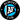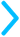Percent and decimal conversions can be confusing.But we've got you covered. Just use our step-by-step convertors!

For both of these conversions, we're just moving the decimal point. If you have trouble remembering which direction to move it in, think of it this way. "D"(ecimal) comes before "P"(ercent) in the alphabet.

### A B C D E F ... M N O P

So, if we're converting from a decimal (D) to a percent (P), we move the decimal point to the right, and if we're converting from a percent (P) to a decimal (D), we move the decimal point to the left.

%